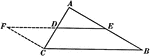Line Joining Midpoints on a Triangle

Illustration used to prove "The line joining the mid-points of two sides of a triangle is parallel to…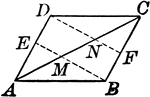Parallelogram With Lines From opposite Vertices to Midpoints

Illustration to show that when lines from two opposite vertices of a parallelogram to the midpoints…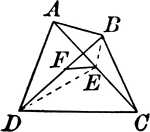Quadrilateral With Diagonals and Midpoints Joined

Quadrilateral with intersecting diagonals and segment connecting midpoints.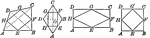Quadrilaterals With Lines Joining Midpoints

Illustrations to show quadrilaterals with lines joining the midpoints of the sides of any quadrilateral,…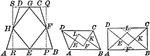Trapezoids With Lines Joining Midpoints

Illustrations to show trapezoids with lines joining the midpoints of the sides of any quadrilateral,…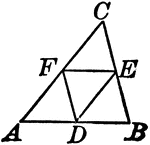Midpoints of Triangle Divide Triangle into Four Equal Triangles

Illustration to show that if the lines joining the middle points of the sides of a triangle divide the…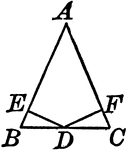Perpendiculars Dropped From Midpoint of Base to Legs of Isosceles Triangles

Illustration to show the perpendiculars dropped from the midpoint of the base to the legs of an isosceles…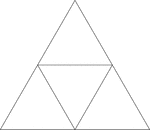Equilateral Triangle Inscribed In An Equilateral Triangle

Illustration of an equilateral triangle inscribed in an equilateral triangle. The smaller triangle is…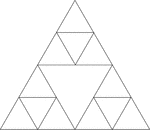Equilateral Triangles Inscribed In An Equilateral Triangle

Illustration of an equilateral triangle inscribed in an equilateral triangle by joining the midpoints…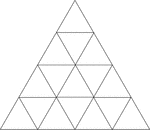Equilateral Triangles Inscribed In Equilateral Triangles

Illustration of an equilateral triangle inscribed in an equilateral triangle by joining the midpoints…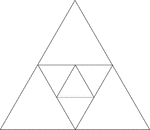Equilateral Triangles Inscribed In Equilateral Triangles

Illustration of an equilateral triangle inscribed in an equilateral triangle by joining the midpoints…